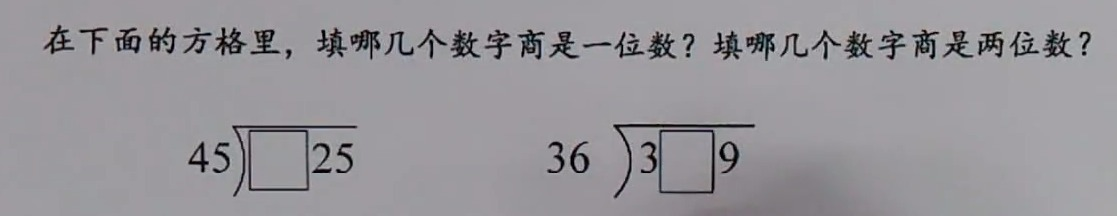10:42:26 四年级 数学 学生：156****392410:59:08 老师：183****6301

• 约等于
• 小于
• 大于
• ÷

除号
• 求积符号
• 并符号
• 交符号
• 属于
• 因为
• 所以
• 垂直于
• 平行线符号
• 弧线
• 波浪号
• 全等于
• 相似于
• 根号
• 小于等于
• 大于等于
• 不小于号
• 不大于号
• 证明号
• ±

正负号
• 求和符号
• ×

乘号
• 对数符号
• 公差符号
• 斜三角形
• 对数符
• 不等于号
• 小于等于
• 小于等于
• 平方米
• 摄氏度
• 公斤符号
• 千分号
• 百分号
• 恒等于
• 立方米
• 无限大号
• 圆周
• 三角形
• 单价
• 积分
• |x|

绝对值

• 约等于
• 小于
• 大于
• ÷

除号
• 求积符号
• 并符号
• 交符号
• 属于
• 因为
• 所以
• 垂直于
• 平行线符号
• 弧线
• 波浪号
• 全等于
• 相似于
• 根号
• 小于等于
• 大于等于
• 不小于号
• 不大于号
• 证明号
• ±

正负号
• 求和符号
• ×

乘号
• 对数符号
• 公差符号
• 斜三角形
• 对数符
• 不等于号
• 小于等于
• 小于等于
• 平方米
• 摄氏度
• 公斤符号
• 千分号
• 百分号
• 恒等于
• 立方米
• 无限大号
• 圆周
• 三角形
• 单价
• 积分
• |x|

绝对值

• 约等于
• 小于
• 大于
• ÷

除号
• 求积符号
• 并符号
• 交符号
• 属于
• 因为
• 所以
• 垂直于
• 平行线符号
• 弧线
• 波浪号
• 全等于
• 相似于
• 根号
• 小于等于
• 大于等于
• 不小于号
• 不大于号
• 证明号
• ±

正负号
• 求和符号
• ×

乘号
• 对数符号
• 公差符号
• 斜三角形
• 对数符
• 不等于号
• 小于等于
• 小于等于
• 平方米
• 摄氏度
• 公斤符号
• 千分号
• 百分号
• 恒等于
• 立方米
• 无限大号
• 圆周
• 三角形
• 单价
• 积分
• |x|

绝对值